What really the “time dilation” in relativity means?

Nimit Theeraleekul B. Eng. (Communication)

(20 Sep 2012)

According to Einstein special theory of relativity, time will run slower in a flying jet plane than it is on the earth. Indeed when a clock was carried in the jet, flying around the world, it seems to shows a slower run of time. Anyway, the time dilation prediction comes from the theory’s mathematical formula which derived from some basic assumptions. So the problem is that the theory tells us nothing about the mechanism behind; why and how the moving clock runs slow!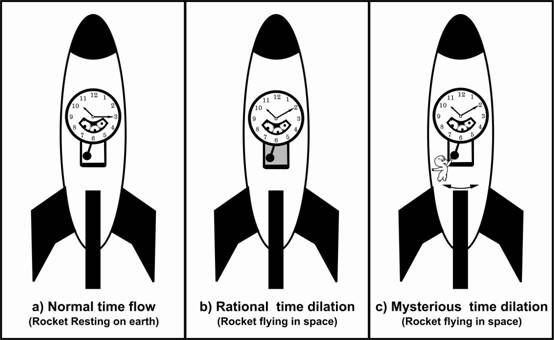Fig.1. Magic vs. realistic mechanism of time dilation

Armed with a new concept of vacuum mechanics (mechanism of vacuum medium) we could improve the theory, which is then makes it possible to explain the mechanism of time dilation. Indeed we will found that it is not real time dilation, instead it is just a slowing of the mechanism of the moving clock!

1) This paper is talking about “the mechanism of time dilation” of any moving clock in which there is no explanation from the conventional interpretation in STR. It is an extension of the paper “Completed Einstein special theory of relativity (CSTR)” present in this website (www.vacuum-mechanics.com). In CSTR we have show how to prove by using a simple scientific experiment (two-solenoid experiment) for the existence of “vacuum medium” instead of the conventional empty space according to STR.

2) First let us start with the three diagrams show in fig.1, which use pendulum clock to show how speed of the clocks was controlled by swinging rate of the pendulum. This will give us an easy way to visualize how to interpret the concept of the mechanism of time dilation when the clock is moving or not!

In (a) the faster swinging rate of the pendulum gives the faster speed of the clock, while the slower swinging rate of the pendulum in (b) gives the slower speed of the clock. The reason behind is because both pendulums were immerged in vacuum medium. So for the flying rocket in (b), the resistive reaction force (of vacuum medium) is greater than what which happened in the resting rocket in (a). It is as if the pendulum in (b) is swinging in viscous fluid, while the pendulum in (a) is not!

3) Now consider fig.1 (c) which represents the conventional time dilation that arisen from mathematical formula derived in STR. And as we have mentioned early that there is no explanation about this time dilation, which indeed was interpreted as a true time dilation, i.e. time itself is slowing. Here, to see how this concept was interpreted, let us follow the explanation by Richard P. Feynman in his famous book “Six not-so-easy pieces: Einstein’s relativity, symmetry, and space-time” as below.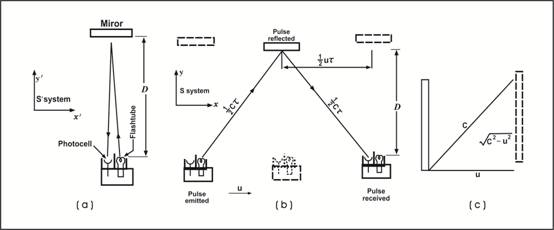Fig.2. A light clock interpretation of time dilation.

In (a) the light clock principle is that when a light signal is keeps going up and down between  the mirrors, making a click each time it comes down, like a standard ticking clock. When another set of the same clock (which was synchronized with the first one) is taking along in a space ship, it is working according to (b), i.e. light moving up and down with a zigzag path as was seen by people at rest on the ground.

In (c) we compare the longer time of the moving light clock (which takes for travelling between the mirrors) with the shorter time of the stationary clock. Therefore the apparent time between clicks is longer for the moving clock, in the same proportion as shown in the hypotenuse triangle. This is how the conventional explanation was done for the time dilation, in which we do not know why the apparent time between clicks which is longer for the moving clock (as observed by one who stay rest) turns out to be a real time dilation!

4) Now we will consider more detail to see how the time dilation mechanism could be explained by using a pendulum clock’s working concept (when the moving mass is increasing) and we will see why it is more rational than the conventional interpretation. First let us return to see how the normal clock (at rest) works as shown in fig.1 (a).

According to diagram fig.3 the simple gravity pendulum is an idealized mathematical model of a pendulum clock closed in vacuum. There is a weight (bob) on the end of a massless cord suspended from a pivot, without friction. When given an initial push, it will swing back and forth at constant amplitude, so it acts as a time keeping device. The period of swing of the pendulum depends on its length, the local strength of gravity; so to a small angle that the pendulum swings away from vertical with a period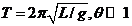.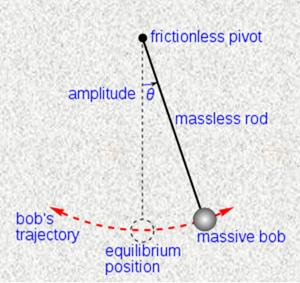Fig.3. A simple pendulum clock.

5) Actually normal pendulum clocks working in air, so there are several ways in which the air changes the measured period, i.e. buoyancy, damping and “added mass” ( Reference: Nelson, Robert; M. G. Olsson (February 1986). “The pendulum – Rich physics from a simple system” American Journal of Physics 54 (2): 112–121.)

Here, what we interested is the case of “added mass”, which corresponds to our consideration, i.e. the increasing mass of a moving bob that occurred when it was immerged in air, in which this equivalent to our case of the moving rocket that the bob is immerged in vacuum medium as shown in fig.1 (b). Anyway, the explanation (in the reference) is rather long and complicate, so we will use the summary explained in Wikipedia encyclopedia, topic “Pendulum; Atmospheric pressure” as follow;

The pendulum carries an amount of air with it as it swings, and the mass of this air increases the inertia of the pendulum, while reducing the acceleration and increasing the period.”

In which, according to our imagination shown in fig.1 (b), it means that the moving bob increase its mass due to the resistive reaction of vacuum medium, which represent by the inertial resistance of the viscous fluid that slow down the pendulum’s swing.

6) Anyway, our first explanation using the pendulum clock because it is simple and easy to visualize. But the problem is that it is not precise enough for real measurement, also its construction is not suitable to use in our case here, in the moving rocket. So a better accurate and practical clock is quartz clock or atomic clock. Here we will talk about quartz clock first as follow.

A quartz clock is a clock which uses an electronic oscillator that is regulated by a quartz crystal to keep time. The crystal will vibrate when applied with electricity and creates a signal with very precise frequency. In crystal which compose of infinite amount of atoms that vibrate harmonically, so we will explain the atomic vibration using chain pendulum by reference to chapter 5 “From Pendulum to Waves and Solitons” in the book “The Versatile Soliton” written by Alexandre T. Fillippov. Here is the summary.

Imagine a periodic sequence of hill and hollow in diagram fig.4 (a), in which atoms rest in the bottom of each hollow and bound by elastic springs. While atoms movement in one layer which were constrained by the neighboring layer shown as the periodic curve (hill and hollow) that placed in a vertical gravitational field, thus the periodic curve visually represents the gravitational potential energy of a single atom.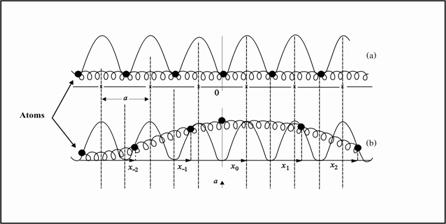Fig.4. Atoms’ harmonic vibration in crystal lattice.

From detail calculation, we could get the final result in mathematical formulas which relate the involved parameters as below;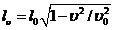…. (1)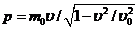…. (2)

[Where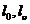= atomic distance at rest and moving respectively,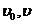= velocity of soliton and acoustic wave respectively,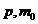= momentum and rest mass of soliton respectively.]

Here we could see that both equation (1) and (2) are in relativistic forms as the conventional relativistic formulas; the only different is acoustic wave instead of light wave. Anyway, armed with our new concept of vacuum medium, we have shown in CSTR that their main principle is the same in this respect!

In conclusion we could see that in crystal lattice, atomic vibration has the relativistic effect in which atomic mass appeared to increase, and the consequent effect is time dilation!

7) Now we will explain the mechanism of time dilation in case of atomic clock which is the most accurate time standard today. The principle of operation of an atomic clock is using the microwave frequency that was emitted when electrons change their energy levels in atoms.

Actually the mechanism of emitting microwave is the same principle as the emitting light wave works (which was explained in “Completed Maxwell electromagnetic field theory” in www.vacuum-mechanics.com ). In summary, when an exited electron jump from unstable excited state back to its normal lower stable state immediately. Because the transition time is very short, then the jumping back electron will transfer its impulse force’s energy to the surrounding vacuum medium. This makes the disturbed vacuum medium oscillates and radiate out as a “wave packet” of the microwave.

Now if an atomic clock is moving, then atoms which generate the standard microwave frequency will subject to the increasing mass effect. And mass of electrons (which change state and generate microwave) also increased, and what follow is their action is slowing which then emit a longer microwave frequency. So the reason that the moving clock runs slower because frequency standard decrease, not time dilate!

8) Finally let us talking about the slowing of disintegration’s time from muons which were used as the evidence of time dilation. Here it is easy to explain its mechanism without using the conventional concept of time dilation; instead it is obvious to use the concept of increasing mass (both from the old STR or our new CSTR) for the explanation.

We know that those muons which come from the cosmic ray traveling to the earth with the great speed, so the increasing of its mass is the answer for the slowing of disintegration’s time. This is because more mass need more disintegration’s time (both the formulas of the increasing mass and time dilation have the same form), which is more rational than the conventional time dilation interpretation!

9) Up to now we could make a final conclusion that the conventional time dilation is an apparent time dilation which occurred by time measurement between any two initial frames moving relative to each other, i.e. it is not a true natural time dilation! Anyway, even time dilation is a relative time, but because the moving clock (which was used to measure time in the moving frame) runs slower due to the dynamic resistance of vacuum medium; this is the mechanism of time dilation!

…………………………..